## 基于python的多因子分析_python 归因分析_尤尔小屋的猫的博客-程序员秘密## 因子分析

### 2种因子分析

• 探索性因子分析：不确定在现有的自变量背后到底有几个因子在起作用，我们通过需要这种方法试图寻找到这几个因子
• 验证性因子分析：已经假设自变量背后有几个因子，试图通过这种方法去验证一下这种假设是否正确。

### 模型推导

z i = a i 1 F 1 + a i 2 F 2 + ⋯ + a i m F m + c i U i ( i = 1 , 2 , ⋯   , p ) z_{i}=a_{i 1} F_{1}+a_{i 2} F_{2}+\cdots+a_{i m} F_{m}+c_{i} U_{i} (i=1,2, \cdots, p)

1、第一点： F j ( j = 1 , 2 , … m ) F_j(j=1,2,…m) 出现在每个变量的式子中且m<p，称之为公共因子

2、第二点： U i ( i = 1 , 2 , … p ) U_i(i=1,2,…p) 仅仅与变量 z i z_i 相关，称之为特殊因子

3、第三点：系数 a i j 、 c i ( i = 1 , 2 … p ， j = 1 , 2 , … m ) a_{ij}、c_i(i=1,2…p，j=1,2,…m) 称之为载荷因子

4、第四点： A = ( a i j ) A={(a_{ij})} 称之为载荷矩阵

z = ( z 1 , z 2 , ⋯   , z p ) T , F = ( F 1 , F 2 , ⋯   , F m ) T , U = ( U 1 , U 2 , ⋯   , U p ) T A = ( a i j ) p × m , C = diag ⁡ ( c 1 , c 2 , ⋯   , c p ) z=\left(z_{1}, z_{2}, \cdots, z_{p}\right)^{T}, F=\left(F_{1}, F_{2}, \cdots, F_{m}\right)^{T}, U=\left(U_{1}, U_{2}, \cdots, U_{p}\right)^{T} A=\left(a_{i j}\right)_{p \times m}, \quad C=\operatorname{diag}\left(c_{1}, c_{2}, \cdots, c_{p}\right)

1.各个特殊因子以及特殊因子和公共因子之间相互独立，即满足：

{ Cov ⁡ ( U ) = diag ⁡ ( σ 1 2 , σ 2 2 , ⋯   , σ p 2 ) Cov ⁡ ( F , U ) = 0 \left\{\begin{array}{l}\operatorname{Cov}(U)=\operatorname{diag}\left(\sigma_{1}^{2}, \sigma_{2}^{2}, \cdots, \sigma_{p}^{2}\right) \\ \operatorname{Cov}(F, U)=0\end{array}\right.

2、各公共因子都是均值为0、方差为1的独立正态随机变量，其协方差矩阵为单位矩阵 I m I_m ，即 F − N ( 0 , I m ) F-N(0,I_m)

3、m个公共因子对第i个变量方差的贡献称之为第i贡献度，记为 h i 2 h_i^2

h i 2 = a i 1 2 + a i 2 2 + ⋯ + a i m 2 h_{i}^{2}=a_{i 1}^{2}+a_{i 2}^{2}+\cdots+a_{i m}^{2}

4、特殊因子的方差称之为特殊方差或者特殊值 σ i 2 , i = 1 , 2 , 3 … p \sigma_{i}^{2},i=1,2,3…p

5、第i个变量的方差分解为： Var ⁡ z i = h i 2 + σ i 2 , i = 1 , 2 , ⋯   , p \operatorname{Var} z_{i}=h_{i}^{2}+\sigma_{i}^{2}, i=1,2, \cdots, p

### 因子载荷矩阵的重要性质

1、因子载荷 a i j a_{ij} 是第i个变量与第j个公共因子的相关系数，反映的是第i个变量和第j个公共因子之间的重要性。绝对值越大，表示相关性的密切程度越高

2、贡献度的统计意义

h i 2 = a i 1 2 + a i 2 2 + ⋯ + a i m 2 = ∑ i = 1 m a i j 2 h_{i}^{2}=a_{i 1}^{2}+a_{i 2}^{2}+\cdots+a_{i m}^{2}=\sum_{i=1}^ma_{ij}^2

Var ⁡ ( X i ) = a i 1 2 Var ⁡ ( F 1 ) + ⋯ + a i m 2 Var ⁡ ( F m ) + Var ⁡ ( ε i ) \operatorname{Var}\left(X_{i}\right)=a_{i 1}^{2} \operatorname{Var}\left(F_{1}\right)+\cdots+a_{i m}^{2} \operatorname{Var}\left(F_{m}\right)+\operatorname{Var}\left(\varepsilon_{i}\right)

3、公共因子 F j F_{j} 方差贡献的统计意义

## 因子分析步骤

• 对所给的数据样本进行标准化处理
• 计算样本的相关矩阵R
• 求相关矩阵R的特征值、特征向量
• 根据系统要求的累积贡献度确定主因子的个数
• 计算因子载荷矩阵A
• 最终确定因子模型

## factor_analyzer库

pip install factor_analyzer


• factor_analyzer.analyze（重点）
• factor_analyzer.factor_analyzer

## 案例实战

### 导入数据

Big Five Model is widely used nowadays, the five factors include: neuroticism，extraversion，openness to experience，agreeableness and conscientiousness.

• 认同性：agree=c(“-A1”,“A2”,“A3”,“A4”,“A5”)
• 勤奋的、有责任的：conscientious=c(“C1”,“C2”,“C3”,“-C4”,“-C5”)
• 外向的：extraversion=c(“-E1”,“-E2”,“E3”,“E4”,“E5”)
• 神经质、不稳定性：neuroticism=c(“N1”,“N2”,“N3”,“N4”,“N5”)
• 开放的：openness = c(“O1”,“-O2”,“O3”,“O4”,“-O5”)

## 导入库

# 数据处理
import pandas as pd
import numpy as np

# 绘图
import seaborn as sns
import matplotlib.pyplot as plt
# 因子分析
from factor_analyzer import FactorAnalyzer


## 数据探索

### 数据信息

df = pd.read_csv("bfi.csv", index_col=0).reset_index(drop=True)
df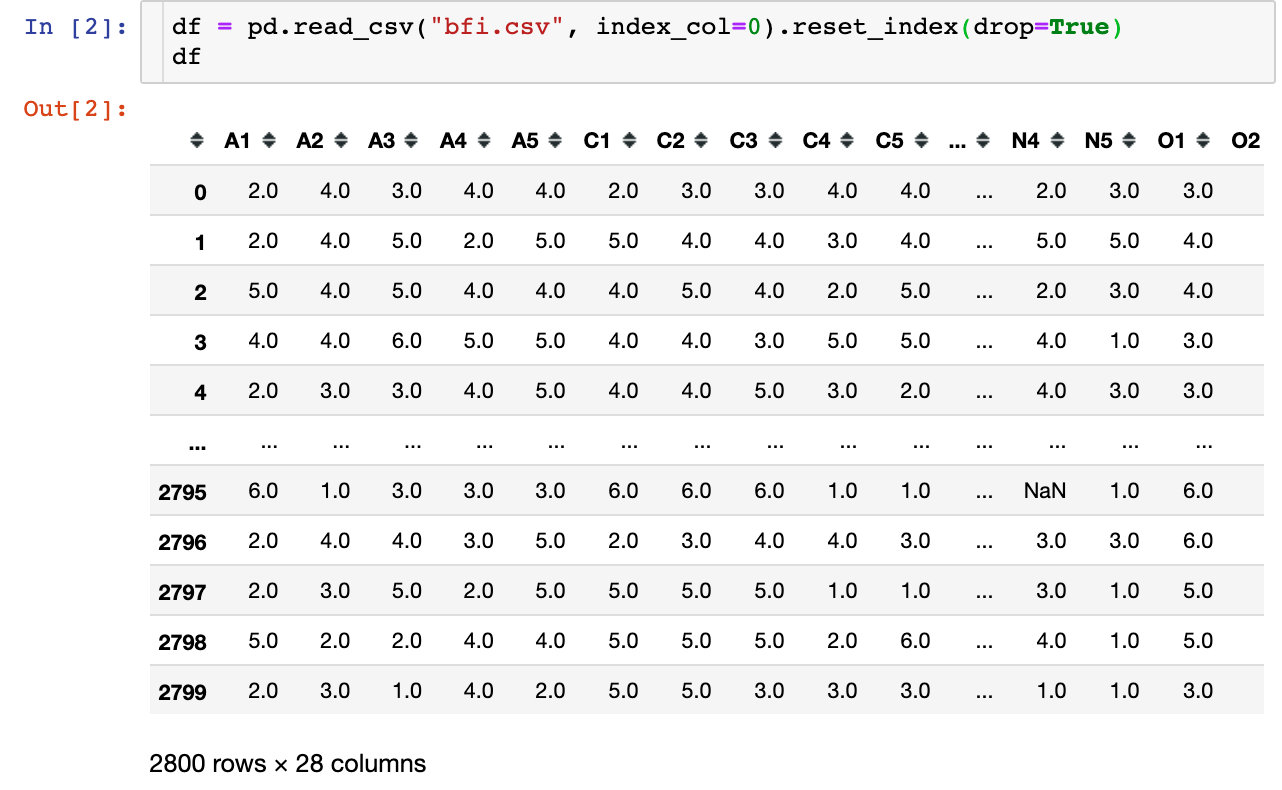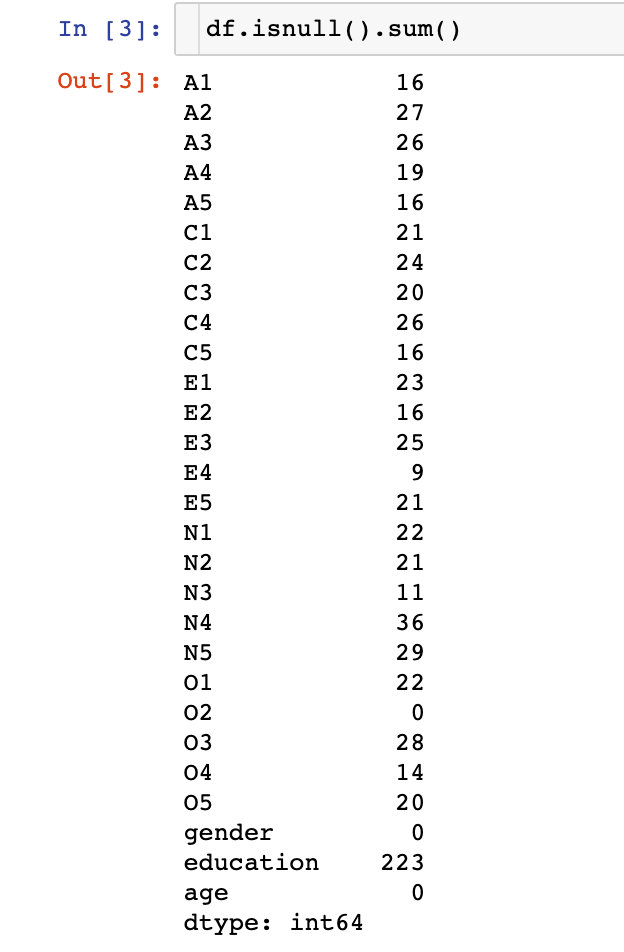### 数据预处理

#  去掉无效字段
df.drop(["gender","education","age"],axis=1,inplace=True)
# 去掉空值
df.dropna(inplace=True)


## 充分性检测

• Bartlett’s球状检验（巴特利球形检验）
• KMO检验

### Bartlett’s球状检验

from factor_analyzer.factor_analyzer import calculate_bartlett_sphericity

chi_square_value, p_value = calculate_bartlett_sphericity(df)
chi_square_value, p_value

# 结果
(18170.96635086924, 0.0)


### KMO检验

Kaiser-Meyer-Olkin (KMO) Test measures the suitability of data for factor analysis. It determines the adequacy for each observed variable and for the complete model.

KMO estimates the proportion of variance among all the observed variable. Lower proportion id more suitable for factor analysis. KMO values range between 0 and 1. Value of KMO less than 0.6 is considered inadequate.

from factor_analyzer.factor_analyzer import calculate_kmo
kmo_all,kmo_model=calculate_kmo(df)
kmo_all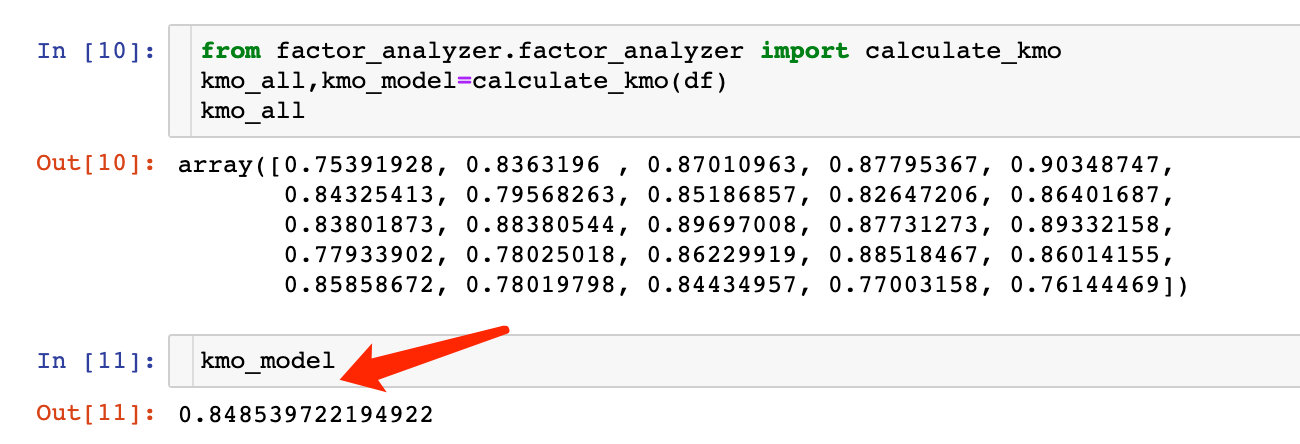KMO大于0.6，也说明变量之间存在相关性，可以进行分析。

## 选择因子个数

### 特征值和特征向量

faa = FactorAnalyzer(25,rotation=None)
faa.fit(df)

# 得到特征值ev、特征向量v
ev,v=faa.get_eigenvalues()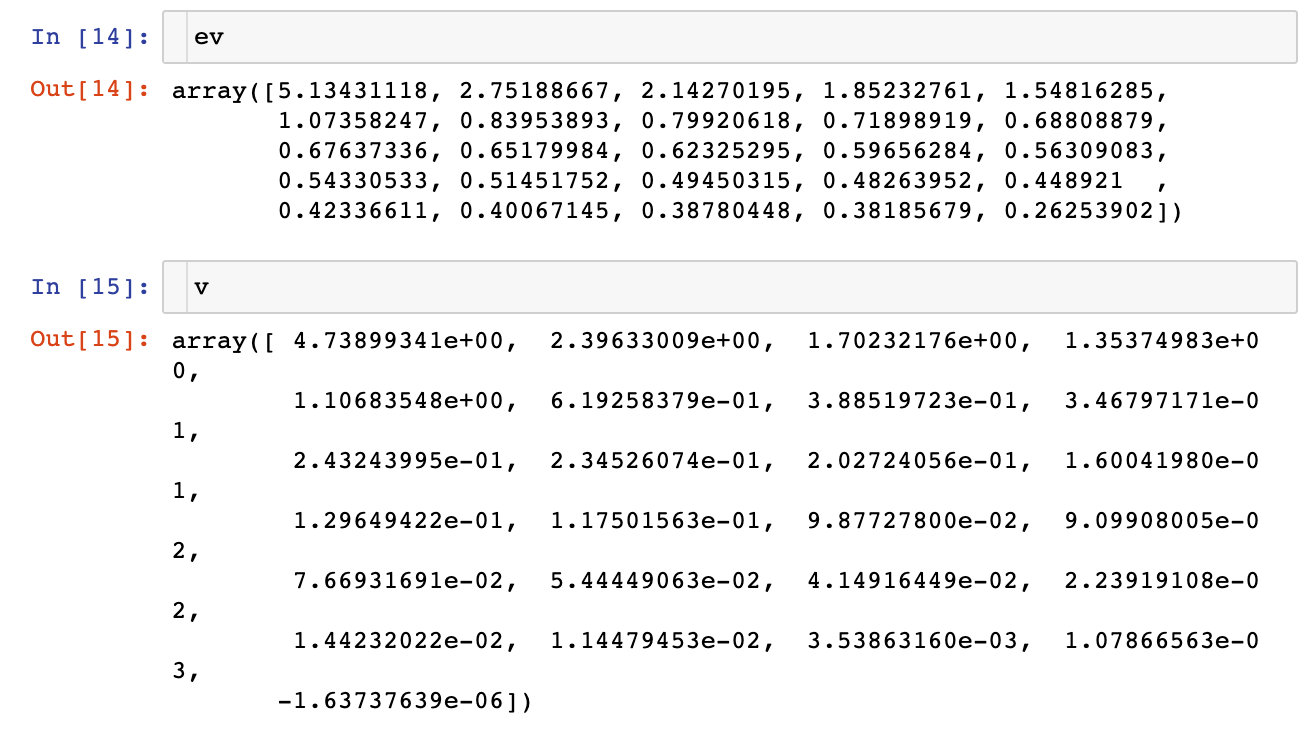### 可视化展示

 # 同样的数据绘制散点图和折线图
plt.scatter(range(1, df.shape + 1), ev)
plt.plot(range(1, df.shape + 1), ev)

# 显示图的标题和xy轴的名字
# 最好使用英文，中文可能乱码
plt.title("Scree Plot")
plt.xlabel("Factors")
plt.ylabel("Eigenvalue")

plt.grid()  # 显示网格
plt.show()  # 显示图形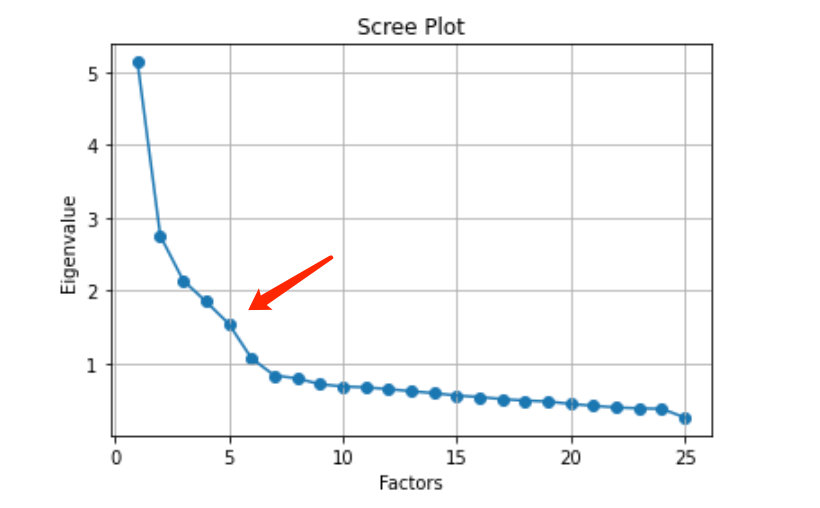## 建模

### 因子分析-fit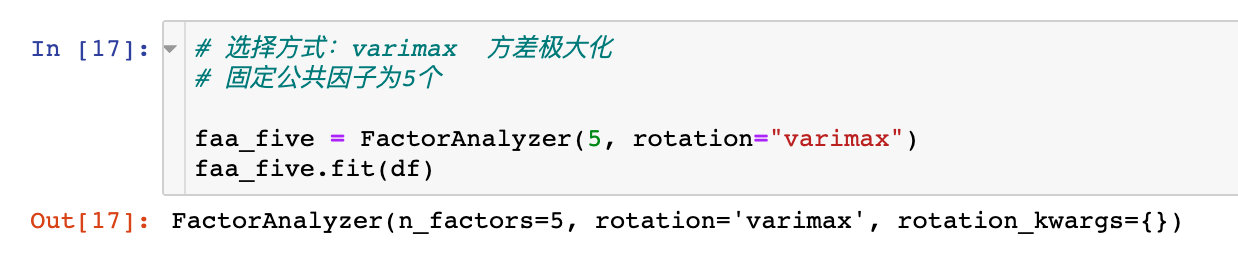ratation参数的其他取值情况：

• varimax (orthogonal rotation)
• promax (oblique rotation)
• oblimin (oblique rotation)
• oblimax (orthogonal rotation)
• quartimin (oblique rotation)
• quartimax (orthogonal rotation)
• equamax (orthogonal rotation)

### 查看因子方差-get_communalities()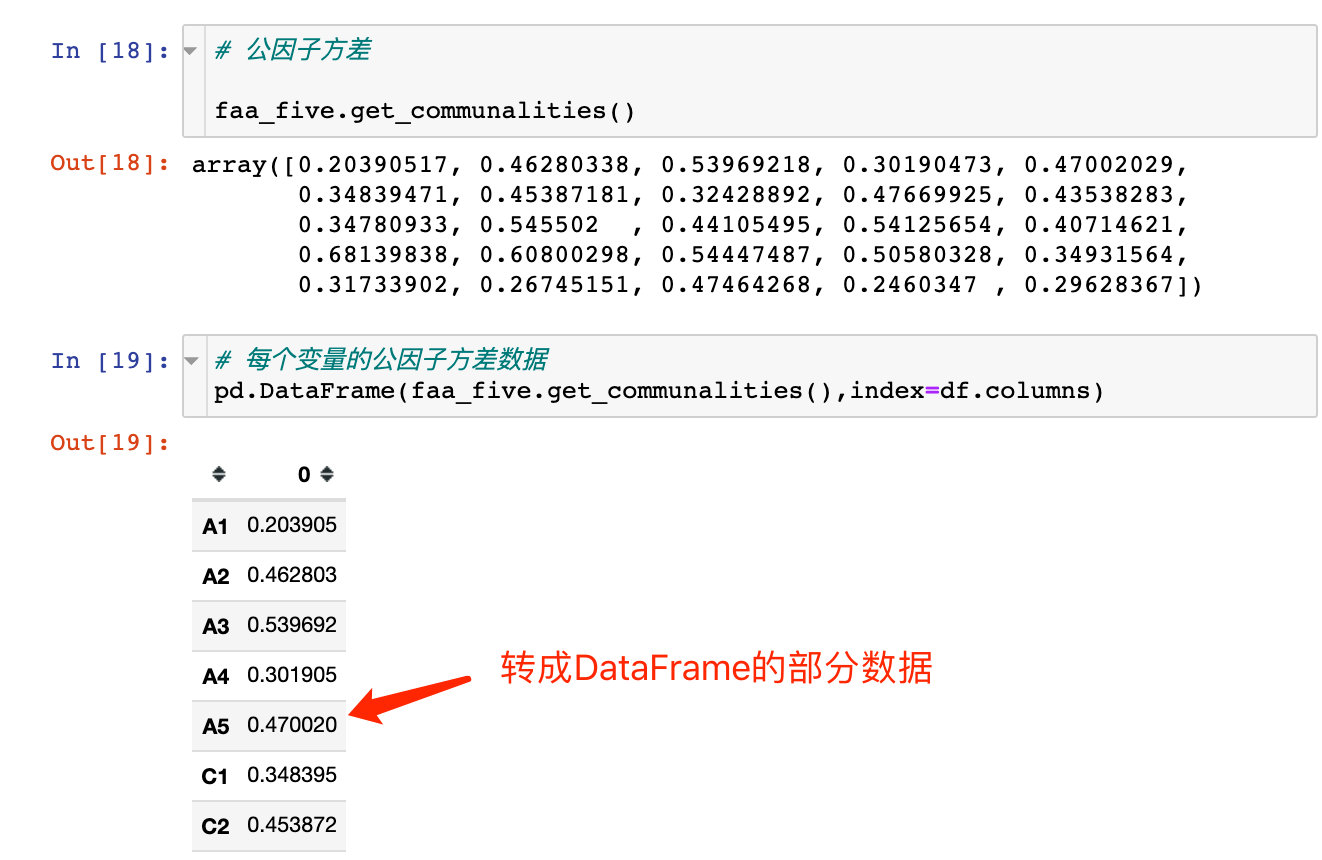### 查看特征值-get_eigenvalues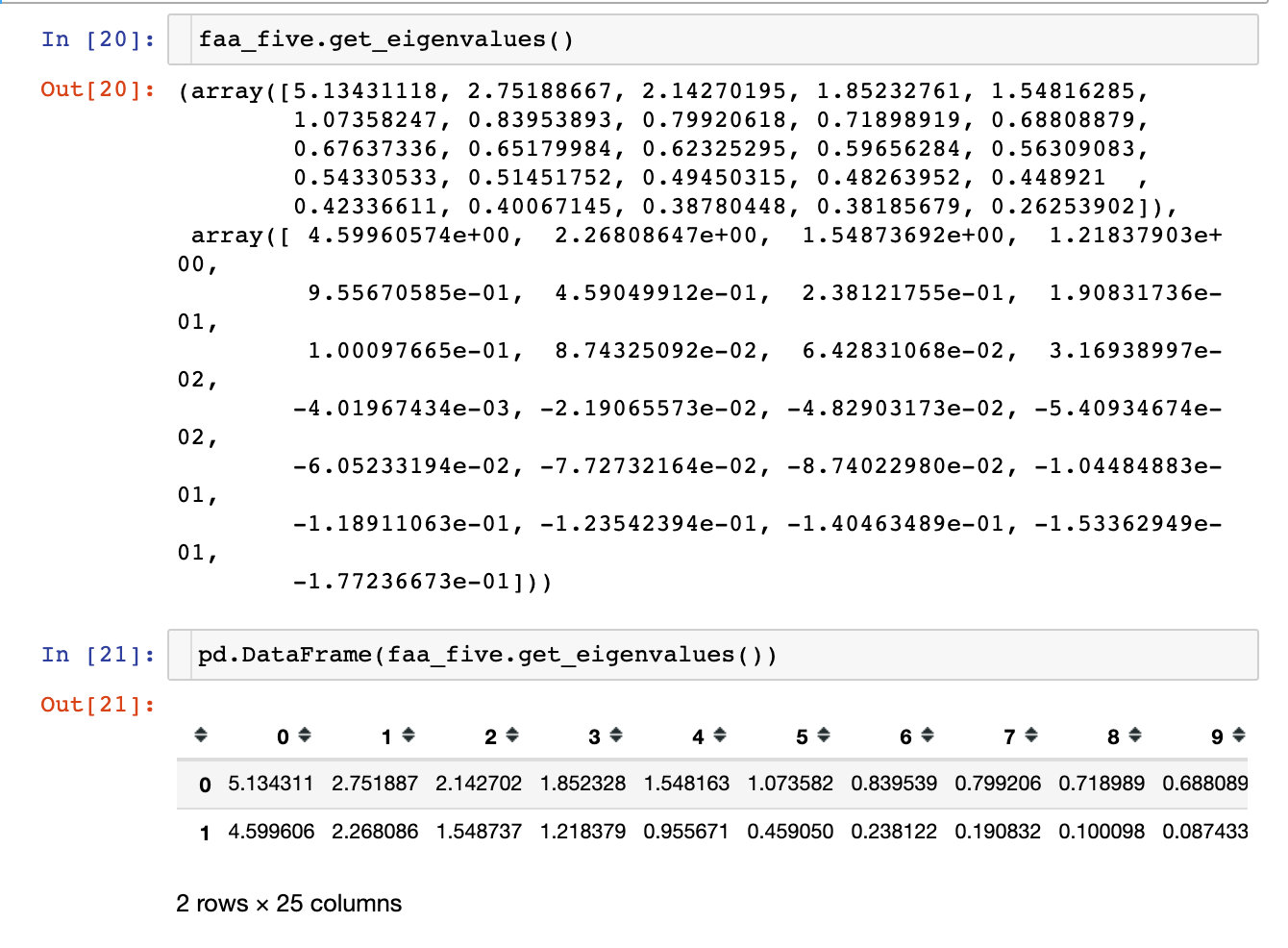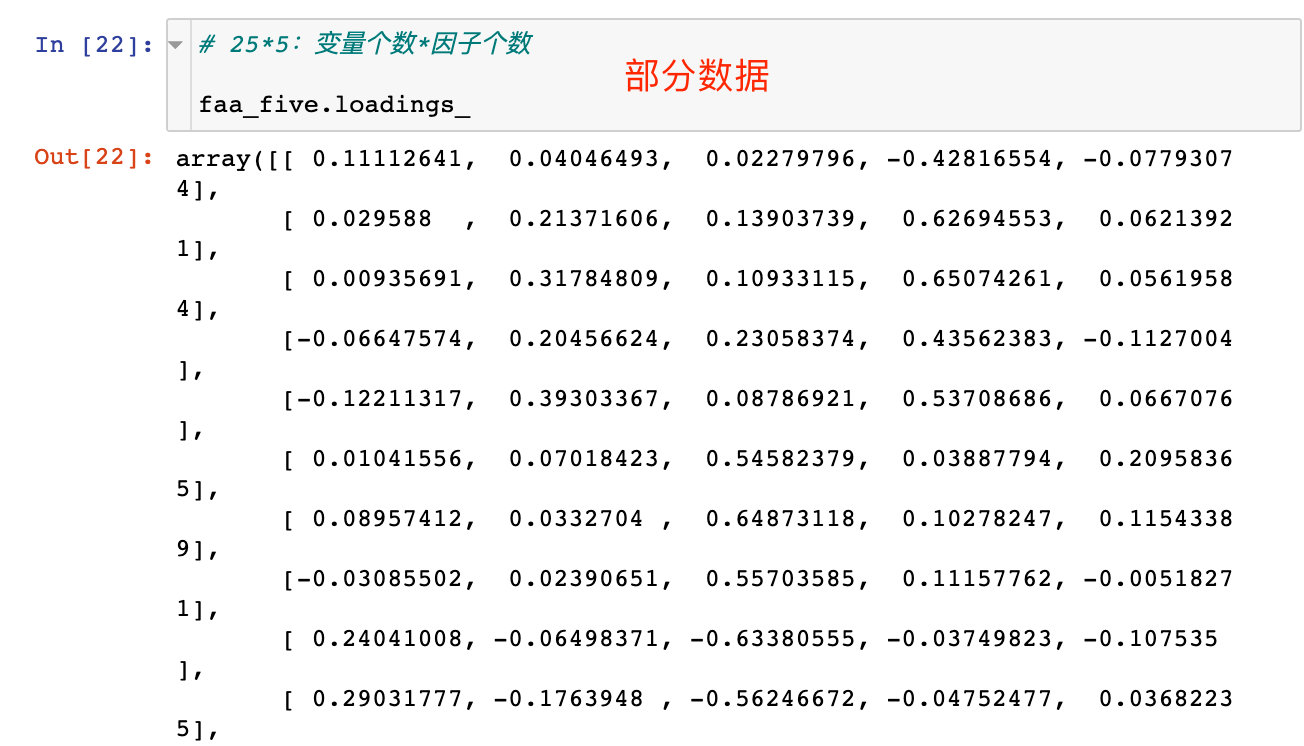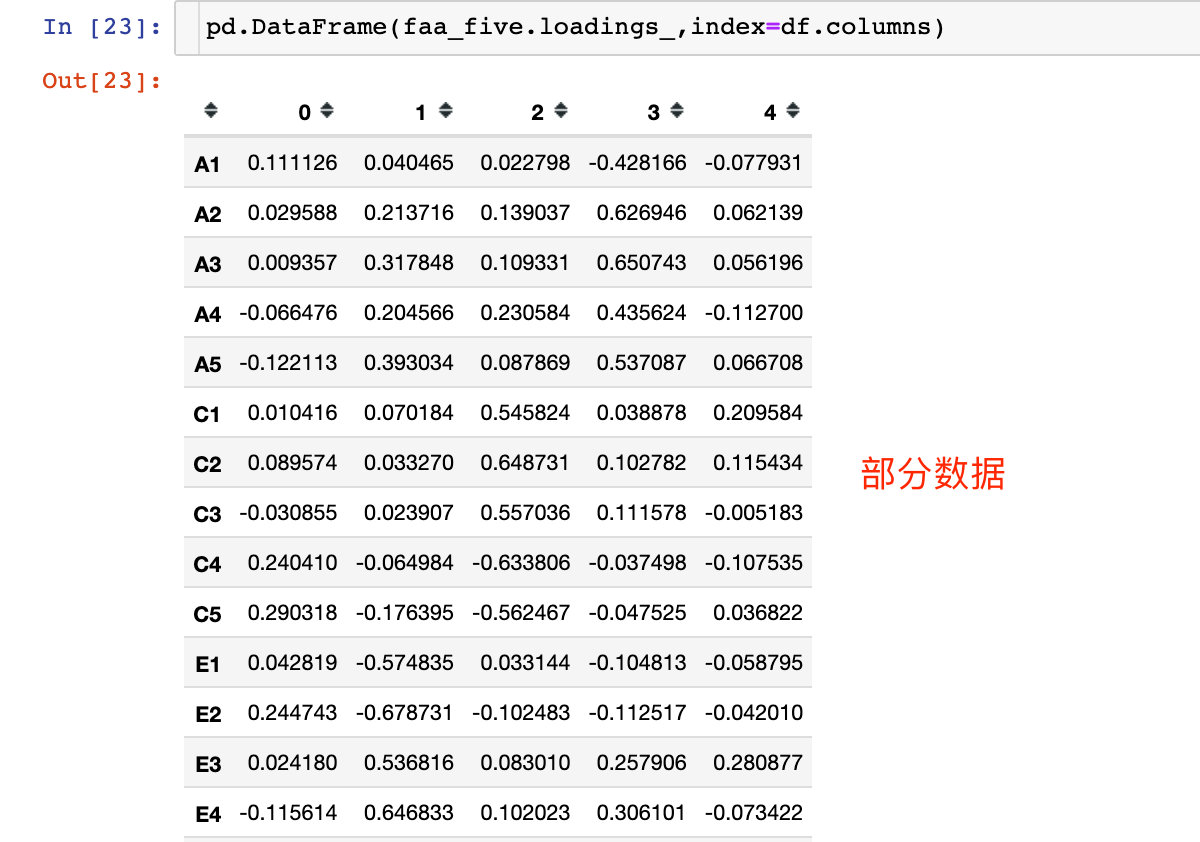### 查看因子贡献率-get_factor_variance()

• 总方差贡献：variance (numpy array) – The factor variances
• 方差贡献率：proportional_variance (numpy array) – The proportional factor variances
• 累积方差贡献率：cumulative_variances (numpy array) – The cumulative factor variances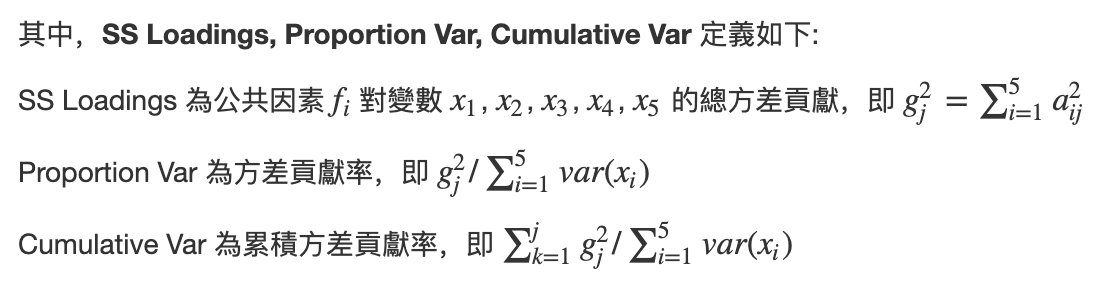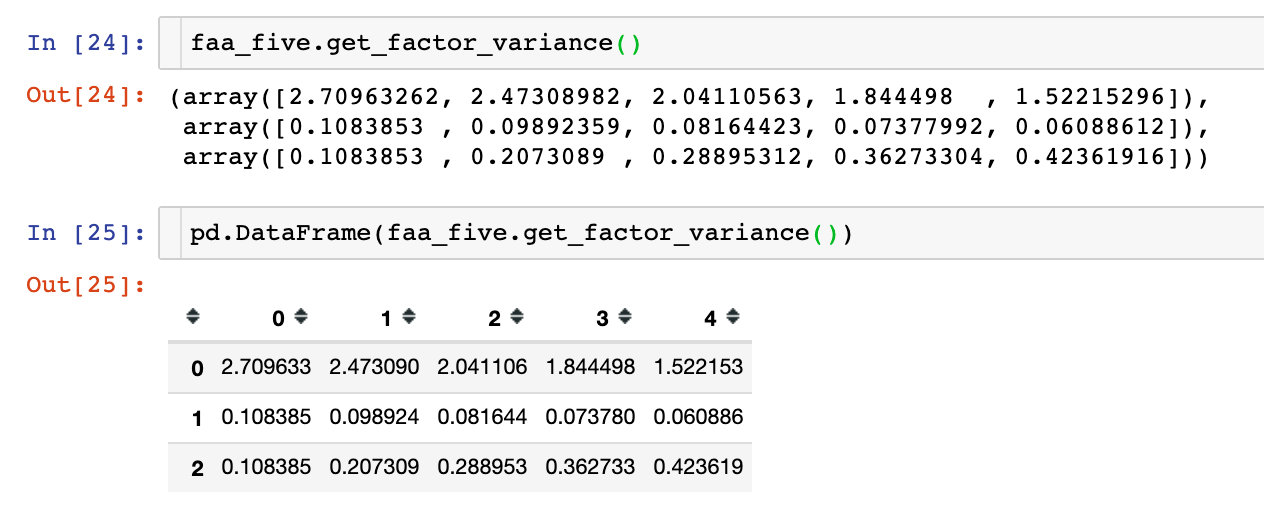## 隐藏变量可视化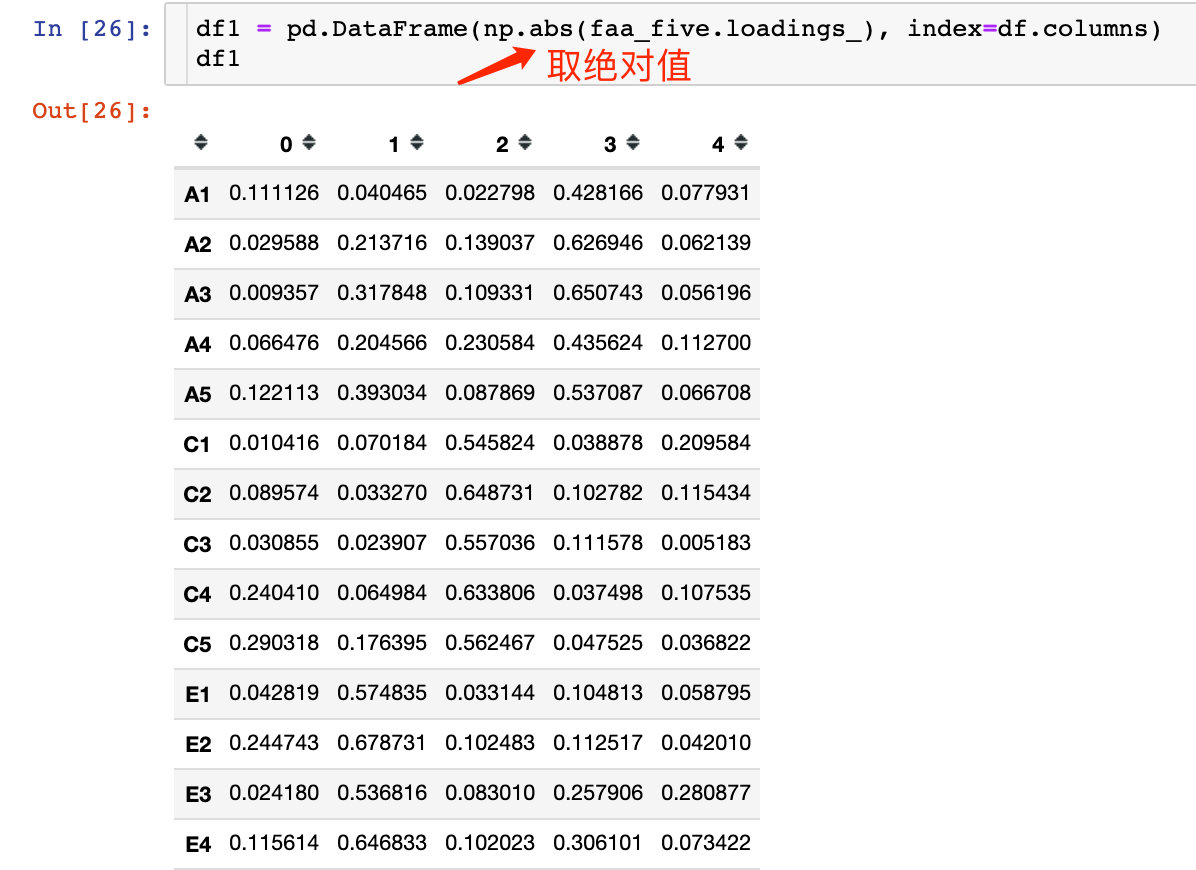# 绘图

plt.figure(figsize = (14,14))
ax = sns.heatmap(df1, annot=True, cmap="BuPu")

# 设置y轴字体大小
ax.yaxis.set_tick_params(labelsize=15)
plt.title("Factor Analysis", fontsize="xx-large")

# 设置y轴标签
plt.ylabel("Sepal Width", fontsize="xx-large")
# 显示图片
plt.show()

# 保存图片
# plt.savefig("factorAnalysis", dpi=500)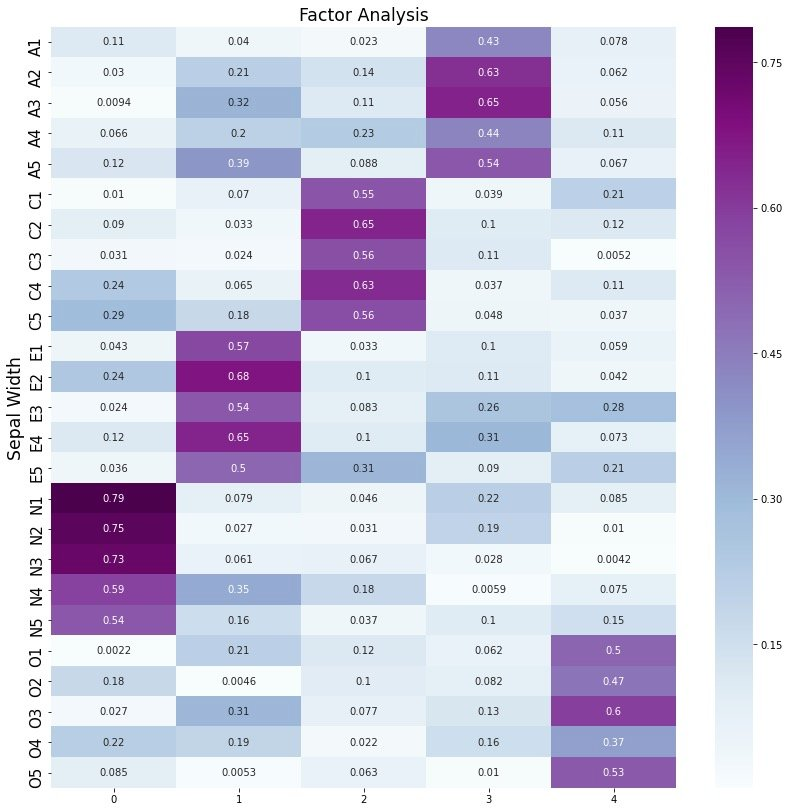## 转成新变量-transformn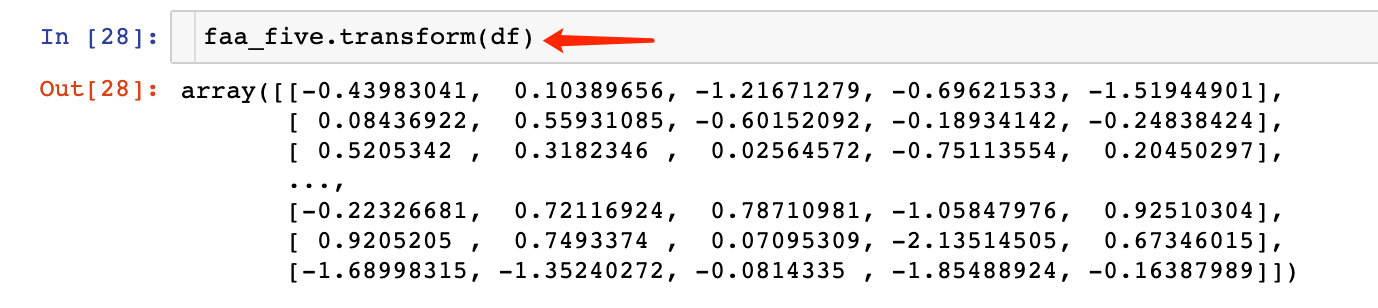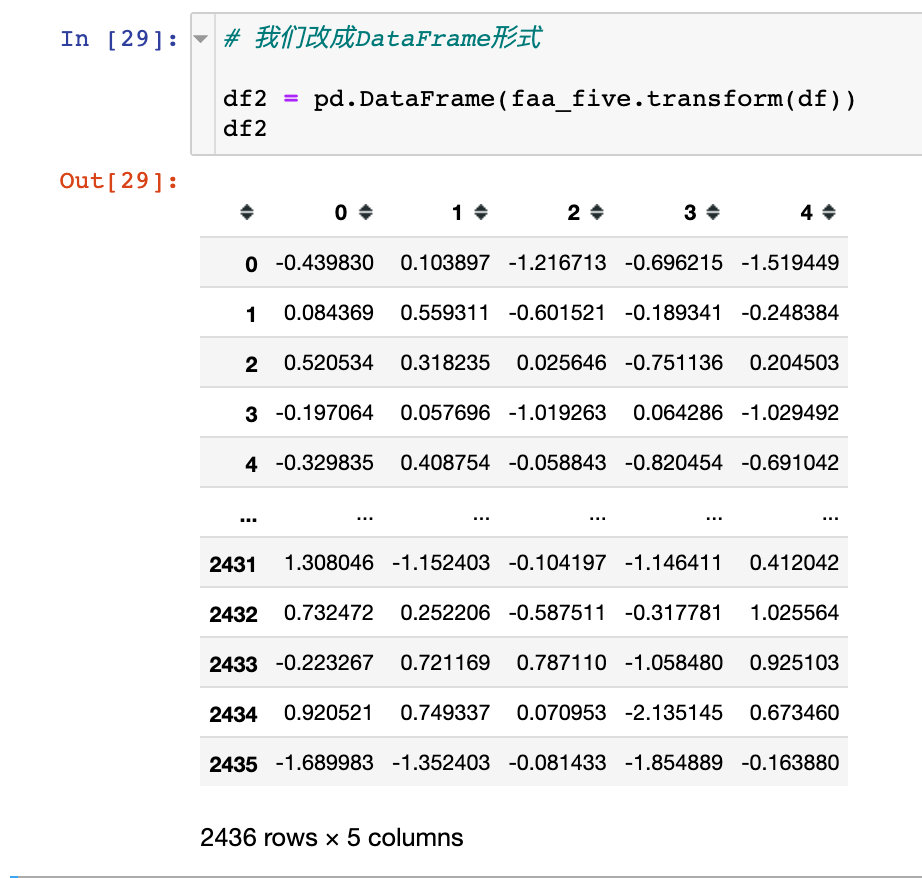1. 原数据的相关性检测
2. 因子个数的探索
3. 因子分析的建模过程
4. 隐藏变量的可视化
5. 转成基于5个新变量的数据

## 参考资料

2、多因子分析：https://mathpretty.com/10994.html

3、factor_analyzer package的官网使用手册：https://factor-analyzer.readthedocs.io/en/latest/factor_analyzer.html

4、浅谈主成分分析和因子分析：https://zhuanlan.zhihu.com/p/37755749

### 忠诚 TYVJ P1038 题解_p1038m spool_five213ddking的博客-程序员秘密

program P1038;const MaxN=100000; type tre=record lc,rc,l,r,mindata:longint; end;var m,n,root,tot:longint; tree:array [-1..MaxN*4] of tre;procedure build(var t:longint;l,r:

### https协议握手过程概述_https的握手做了哪些事情_土豆爸爸的博客-程序员秘密

https是使用了SSL的http，也就是说主要是多了加密这一块。 https其实有两个功能：1，身份验证2，数据加密只是说身份验证可以认为是数据加密需要的过程，但是不妨这是一个很重要的功能，具体握手过程为：(流程都是根据网上搜索个人组织整理的，如果有不完整不完善的请留言) 客户端发起tcp三次握手，正常的传输层建立连接；客户端发送自己的ssl版本号，支持的加密...

### NLP：LSTM之父眼中的深度学习十年简史《The 2010s: Our Decade of Deep Learning / Outlook on the 2020s》的参考文献_一个处女座的程序猿的博客-程序员秘密

Paper：LSTM之父眼中的深度学习十年简史《The 2010s: Our Decade of Deep Learning / Outlook on the 2020s》的参考文章The 2010s: Our Decade of Deep Learning / Outlook on the 2020sReferences Beyond Those in Reference[MIR...